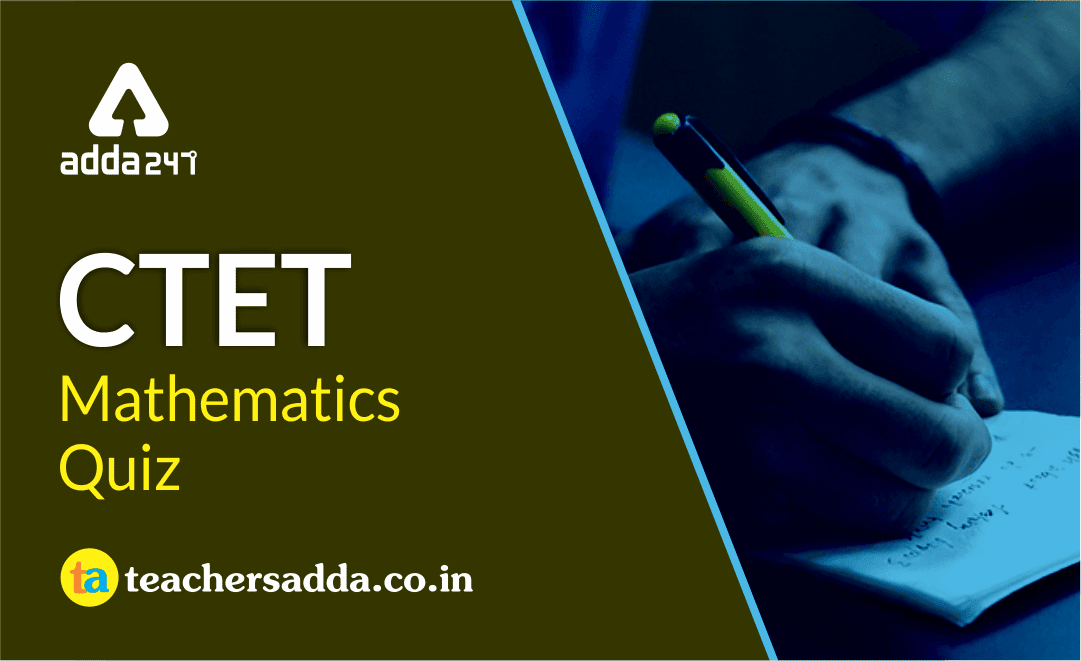Dear Students!!! There is most general as well as a scoring section in all the competitive entrance examinations in the teaching field i.e "Mathematics".Because in this section only one thing is work i.e your accuracy and that could be nourished with the daily practice. So, for this, we are providing you the daily quiz for all teaching exams i.e CTET Exam 2019DSSSB ,KVS,STET Exam.

Q1. Misconceptions are incorrect understanding of mathematical concepts. How can a teacher help her students with their misconceptions?
By observing their work and talking to them to know their understanding
By suggesting some good books for practice
By demonstrating more examples of correct procedures followed by revision
By not interfering at all as children will learn eventually

Q2. When children were asked to compare fractions, a student compared only the numerators and considered the one having larger numerator as the larger fraction. This suggests that the child
Is irresponsible
Does not know the concept of fractions
Has not practiced well
Has not understood the idea of magnitude of fractions

Q3. A good strategy to help students with their misconceptions can be
Demonstration of correct procedure
Encouraging practice and drill
Giving non-examples
Posing questions

Q4. When teaching addition of fractions, a teacher came across the following errorWhat remedial action can the teacher take in such a situation?

Suggest lots of books to practice
Help children understood the need to make the denominators equal
The child will, eventually, learn the idea; so, there’s no need to intervene
Ask the parents to give more attention on child’s education

Q5. A common error that we often encounter when children are asked to add two fractions is: they add the numerator with the numerator and denominator with the denominator. What could be a possible reason for such a misconception ?
Lack of understanding of the teacher
Lack of attention
Prior understandings of children related to adding whole numbers
Non-availability of teaching–learning material

Q6. The area of a right angled triangle ABC, right angled at B, is 46 sq units. A median is drawn from A to BC which intersects at D. Find the area (in sq. units) of triangle ABD.
12
23
46
88

Q7. X and Y give an exam. Difference of their marks is 45 and the difference of their marks is 15% of the maximum marks. What is the maximum marks for the exam?
180
450
150
300

Q8. If P : Q : R = 6 : 7 : 8 and R : S = 3 : 5, then what is P : S?
18 : 25
9 : 20
11 : 24
16 : 45

Q9. In what ratio must a mixture of 11% sugar strength be mixed with that of 25% sugar strength so as to get a new mixture of 13% sugar strength?
5 : 1
6 : 1
7 : 2
10 : 3

Q10. What is the average of 90, 250, 240, 204, and 616?
280
210
230
255